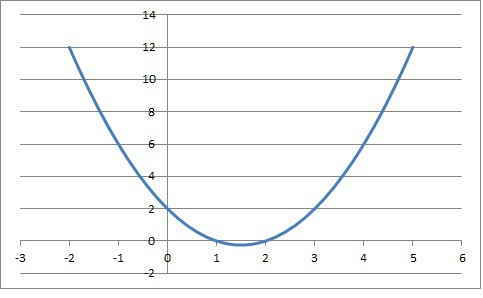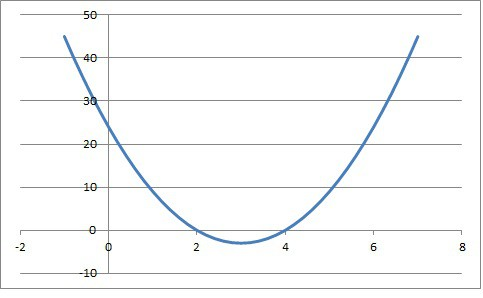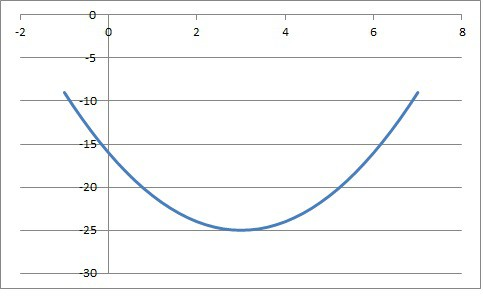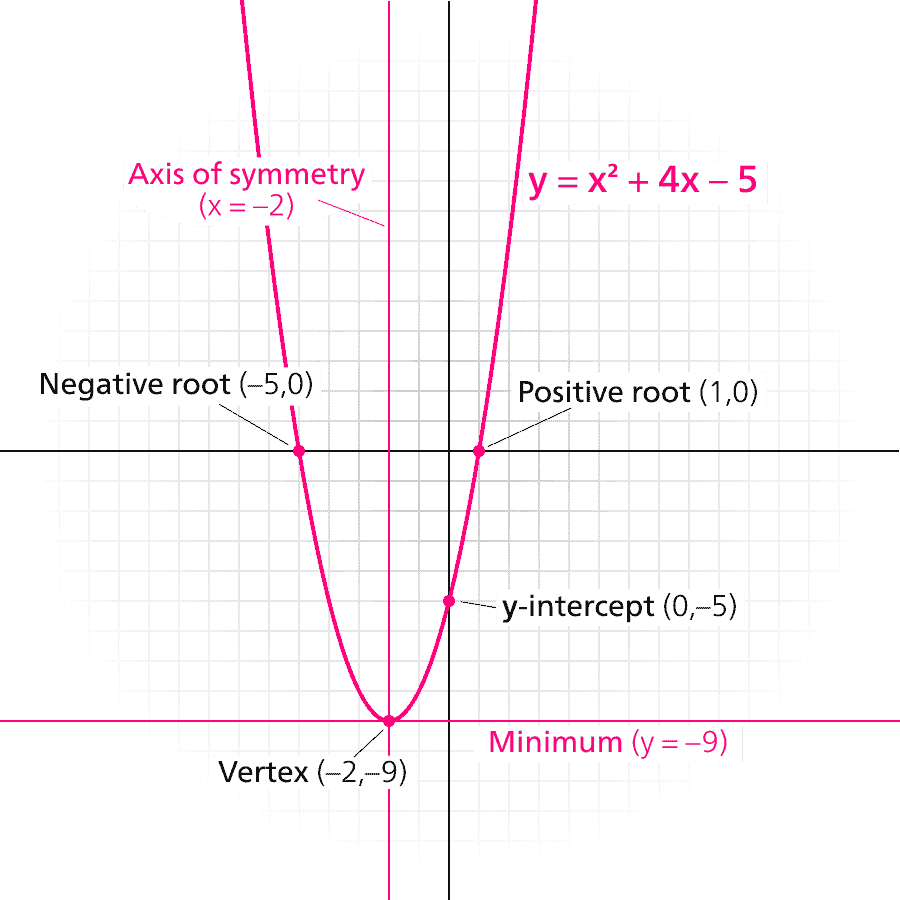# What Is A Quadratic Function? (3 Key Things To Know)

Quadratic functions are often used in mathematics, especially when solving quadratic equations by graphing.  If you are wondering exactly what a quadratic definition is, I have a simple definition for you.

So, what is a quadratic function?  A quadratic function is a function whose standard form has the equation f(x) = ax2 + bx + c, where a is not zero.  If you graph a quadratic function, you get the shape of a parabola.  A quadratic function can have two distinct real zeroes, one repeated real zero, or two complex zeroes.

Of course, a quadratic function can also intersect other shapes in a coordinate plane.  We can find the points of intersection by setting the ‘y’ variables equal for the two shapes.

In this article, we’ll talk about quadratic functions and the 3 forms you might see.  We’ll also talk about how to solve quadratic function and see what they look like when you graph them.

Let’s get started.

## What Is A Quadratic Function?

A quadratic function has the standard form f(x) = ax2 + bx + c, where a is not zero (if a equals zero, then the function is linear, not quadratic!)

When we graph a quadratic function with x as the input (independent variable) and y = f(x) as the output (dependent variable), we get the shape of a parabola. Some parabolas will be even functions.

When Does A Quadratic Have No Solut...
When Does A Quadratic Have No Solution?

We mentioned the standard form of a quadratic equation above, but there are two other forms to be aware of.

## What Are The 3 Forms Of A Quadratic Function?

There are three different forms for a quadratic equation, but they are all equivalent and look the same when you graph them.  They are:

• Standard Form – this is the most common form, and it is helpful when we solve using the quadratic formula.
• Factored Form – this form tells you the factors of the quadratic, and we can easily find the zeros from there.
• Vertex Form – this form tells you the vertex (highest or lowest point) of the parabola that results from graphing a quadratic function.

Let’s take a closer look at how to write a quadratic function in each of these three forms, along with some examples.

### Standard Form Of A Quadratic Function

The standard form of a quadratic function looks like this:

• f(x) = ax2 + bx + c, where a is not equal to zero.

With this form, we can easily tell whether the graph of the parabola opens up (a > 0) or down (a < 0).

We can also rearrange standard form into other forms by factoring or by finding the vertex.

Let’s take a look at an example of a quadratic function in standard form.

#### Example: Standard Form Of A Quadratic Function

If we have the quadratic function

• f(x) = x2 – 3x + 2,

then it is already in standard form, with a = 1, b = -3, and c = 2.

We can convert this standard form to factored form by factoring the right side:

• f(x) = (x – 1)(x – 2)  [-1 and -2 have a sum of -3 and a product of +2]

Note that after factoring, it is easy to see the zeros of the function: x = 1 and x = 2.  These are the values of x we would plug into f(x) to get an output (y-value) of zero.

If we find the vertex, we can also switch to vertex form.

Remember that the x-coordinate of the vertex of a parabola is x = -b/2a.  In this case, we get x = -(-3) / 2(1) = 3/2 or 1.5.

Note that the x coordinate of the vertex is halfway between the two zeros (that is, 1.5 is halfway between 1 and 2).  This is always true, due to the symmetry of a parabola.

Plugging x = 1.5 into f(x), we get y = f(1.5) = -0.25.

That means the vertex of the parabola has coordinates (1.5, -0.25).

The vertex form of a parabola is y = a(x – h)2 + k, where (h, k) is the vertex and a is the same as in the standard form.

Here, we have (h, k) = (1.5, -0.25), so we plug in h = 1.5, k = -0.25, and a = 1 to get:

y = a(x – h)2 + k

y = 1(x – 1.5)2 + -0.25  [h = 1.5, k = -0.25, a = 1]

f(x) = (x – 1.5)2 – 0.25  [y = f(x)]

So, the vertex form of this quadratic function is f(x) = (x – 1.5)2 – 0.25.

The graph is pictured below.The graph of the quadratic function f(x) = x2 – 3x + 2.

Note that this function has repeating y values.

### Factored Form Of A Quadratic Function

After converting from the standard form, the factored form of a quadratic equation looks like this:

• a(x – d)(x – e),

where d and e are the zeros of the function f(x).

The value of a is the same as in standard form, so all we need to do is solve the quadratic x2 + (b/a)x + (c/a) = 0 to find the values of d and e for factored form.

#### Example: Factored Form Of A Quadratic Function

• f(x) = 3x2 – 18x + 24

First, we can factor out a = 3 to simplify the quadratic to

• f(x) = 3(x2 – 6x + 8)

Then, we can factor the quadratic (-2 and -4 give a sum of -6 and a product of +8):

• f(x) = 3(x – 2)(x – 4)

So, this is the factored form for the quadratic.  It is easy enough to switch to vertex form.

Remember that once again, the x-coordinate of the vertex is half way between the zeros of the quadratic function.  Here, the zeros are x = 2 and x = 4 (since the factors are x – 2 and x – 4).

So, the x-coordinate of the vertex is x = 3 (halfway between x = 2 and x = 4).

Plugging this x-value into f(x) gives us the y-value of the vertex: f(3) = -1.

Then the vertex (h, k) is (3, -1).

We already know that a = 1, so vertex form is:

y = a(x – h)2 + k

y = 3(x – 3)2 – 1  [a = 3, h = 3, k = -1]

f(x) = 3(x – 3)2 – 1    [y = f(x)]

The graph is pictured below.The graph of the quadratic function f(x) = 3x2 – 18x + 24.

### Vertex Form Of A Quadratic Function

If we start off with the vertex form of a quadratic function, we can FOIL and then combine like terms to change it to standard form.

We can then then factor or use the quadratic formula to find the zeros and put it into factored form as we did before.

Let’s take a look at an example of how this would work.

#### Example: Vertex Form Of A Quadratic Function

Let’s say we have the vertex form of a quadratic equation:

• f(x) = (x – 3)2 – 25

It is easy enough to FOIL (x – 3)2 = (x – 3)(x – 3) as x2 – 6x + 9.  Then we get:

• f(x) = (x2 – 6x + 9) – 25
• f(x) = x2 – 6x – 16

Now that we have the quadratic function in standard form, we can also factor it to find factored form:

• f(x) = (x – 8)(x + 2)  [-8 and 2 have a sum of -6 and a product of -16]

The zeros of the quadratic function are easy to find from factored form: they are x = 8 and x = -2.

The graph is pictured below.The graph of the quadratic function f(x) = x2 – 6x – 16.

## How Do You Know If A Function Is Quadratic?

A quadratic function will have degree 2.  That is, the highest power of the variable x in the equation will be 2, as in these functions:

• f(x) = x2 – 4x + 4
• f(x) = 3x2 + 7x + 1
• f(x) = -8x2 – 12x + 16
• f(x) = x2 + 5x  [this is a quadratic function with a zero constant term]
• f(x) = x2 – 25  [this is a quadratic function with a zero ‘x’ term]

A higher power of x would mean a different type of function.  For example, a highest power of 3 would be a cubic function, such as f(x) = x3 – 5x2 + 4x – 7.

A highest power of 4 would be a quartic function, such as f(x) = x4 – 4x3 + 9x2 – 8x – 2.

A lower power of x would mean that we are looking at a linear function, such as f(x) = 2x – 3.

### What Is The Difference Between Linear & Quadratic Functions?

A linear function has a zero coefficient for its x2 term and is graphed as a line.  On the other hand, a quadratic function has a nonzero coefficient for its x2 term and is graphed as a parabola.

Both linear and quadratic functions can have either a zero or nonzero ‘x’ coefficient and either a zero or nonzero constant term.

The table below summarizes the difference between linear and quadratic functions.

## What Does A Quadratic Function Look Like?

A quadratic function looks like a parabola when we graph it.  This means that is has a vertex at the lowest point (for a > 0) or the highest point (for a < 0).

This vertex has an x-coordinate of –b/2a.  If we draw a vertical line through this point (with equation x = -b/2a), then we will see “mirror images” on both sides of the line.

That is, the vertical line x = -b/2a “cuts the parabola in half”, splitting the graph into two symmetric halves that are mirror images of one another (when reflected across the line x = -b/2a).

Below you can see the graph of the parabola from the quadratic function y = x2 – 4 + 5.The graph of the parabola from the quadratic function y = x2 – 4 + 5.

## How To Solve A Quadratic Function

When you are asked to solve any equation, you must find the values of variables that make the equation true.

To solve a quadratic function, we must first decide on the value of y (also known as f(x)) that we want to find.  (Remember that the quadratic function will never equal a particular y value if it that y value is below the vertex for a > 0 or above the vertex for a < 0).

Next, we must set the quadratic function equal to that y value.

Then, we rearrange the resulting quadratic equation to put it in standard form.

Finally, we solve the quadratic in standard form using factoring, graphing, or the quadratic formula.

There are three possible cases for the solutions of a quadratic equation:

Let’s look at an example of how to solve.

### Example: Solving A Quadratic Function

Let’s say we have the quadratic function f(x) = x2 + 6x + 7.  I want to find out when this function has a value of 2 (that is, I want to find the x-values that will give us a y-value of 2).

Remember that y = f(x), so we really want to solve y = x2 + 6x + 7 when y = 2:

• y = x2 + 6x + 7  [the original quadratic function]
• 2 = x2 + 6x + 7  [substitute y = 2]
• x2 + 6x + 5 = 0  [change to standard form of a quadratic equation]
• (x + 1)(x + 5) = 0  [factor]

So the solutions are x = -1 and x = -5.

We can check these values in the original equation to find that both f(-1) = 2 and f(-5) = 2.

## Conclusion

Now you know what a quadratic function is and how to recognize the 3 forms.  You also know how to solve quadratics and what the graph looks like when you draw it.

You might also want to check out my article on quadratic sequences.

This article goes into detail on how to use a quadratic to find the nature of the solutions (real or complex) of a cubic function.

If you really want to delve deep, you can learn about quintic functions in my article here or my article on quartic functions here.

I hope you found this article helpful.  If so, please share it with someone who can use the information.

~Jonathon